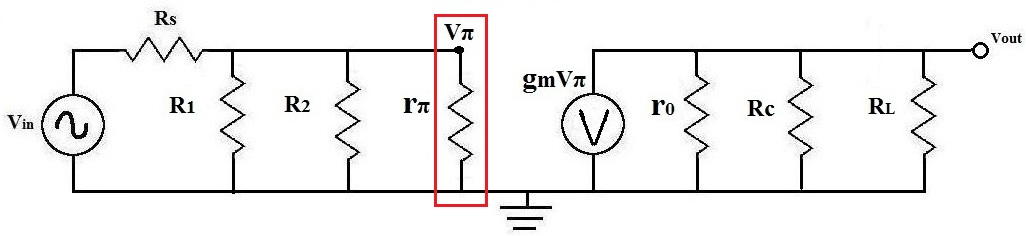﻿ What is Vπ (Vpi) of a Transistor?

# What is Vπ (Vpi) of a Transistor?

Vπ is the voltage that drops across the resistor, rπ, of a transistor.When doing AC analysis, Vπ is an important calculation to make because it is the voltage in parallel with the current amplifier and the output resistors. The amplified current signal of a transistor is usually calculated by the formula I=gmVπ, so it is important to know how to find the value of Vπ.

To find out how to calculate Vπ, read How to Calculate Vπ of a Transistor. 